# Decipede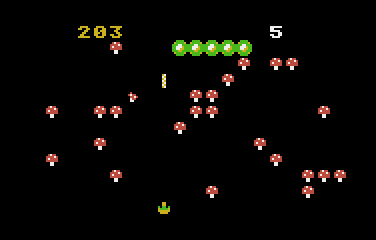This is a little game for the 2017 NOMAM's BASIC 10-liners Contest. This program fits in the EXTREM-256 category, and it was written using TurboBASIC XL 1.5 for the 8-bits ATARI XL/XE. Development started on 2017-02-12, and it took 2+5 days with Kevin Savetz. The final version's date is 2017-04-03.

UPDATE: It obtained the 2nd place of 12 entries in the category.

## Description

Kill the caterpillars before they reach the bottom of the garden. Be careful! They can eat you.

## Instructions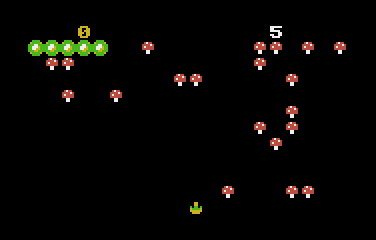When the game starts, your player is located at the bottom of the garden. There are many mushrooms in it. A caterpillar will appear at the top.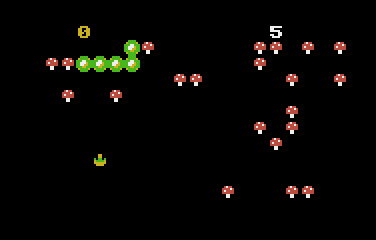Use the joystick to move around in the bottom of the screen and avoid to be eaten by the caterpillar.Use fire button to shoot at the caterpillar.Every time you hit it, the caterpillar might shrink a bit or split in two mini caterpillars.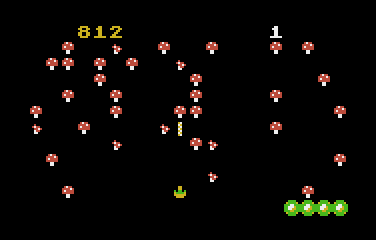Mushrooms appear every time you hit the caterpillar, and when the caterpillar steps down, when it goes away, or when you clear a row of mushrooms.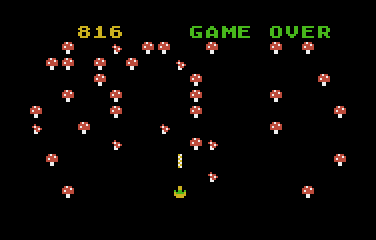The game is over when the caterpillar eats you or when 5 caterpillars go away.

## Development of the game

This is the second part of the story I wrote in Minipede.

When Kevin told me that he was satisfied with Minipede instead of trying the splitting version again, I was convinced that something interesting could be done.

The slow down between one and two caterpillars versions was a fact. The code was the same for both prototypes, but in the seccond one I added a FOR-NEXT loop to manage both caterpillars, one of it at a time, half of the speed!!! But there was some extra code inside the loop: a debugging message in the top of the playfield.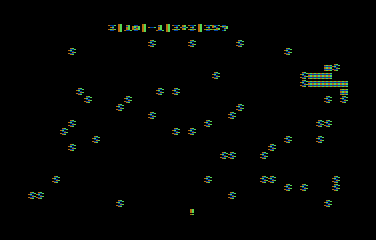New single caterpillar prototype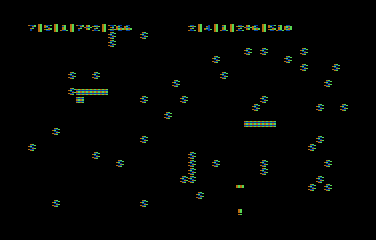New multiple caterpillar prototype

It is well known that the PRINT routine is slow, specially if it needs to convert numbers from the internal BCD structure of numeric variables into user-readable digits, and the debug messages had 6 of them for each caterpillar. I removed the PRINTs and the result was amazing. I could set it up to show 4 caterpillars at the same time.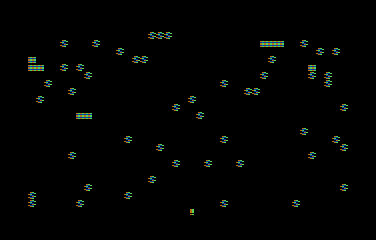Multiple caterpillar prototype with 4 segments

But 4 segments was not so speedy, and 3 segments was acceptable if I had to add the code for the collision of the missile with the caterpillar. The base code for the prototypes only included the code for the movement of the player's gun and the missile.

3 segments, 3 segments... Hum... A caterpillar with 10 pieces could be splitted into 5 segments. How many pieces should the caterpillar have to be splitted to a maximum of 3 segments? Let's see: 3 one-piece segments and 2 destroyed segments. That is 5 pieces. What about 6 pieces? Two splits could result in 2+1+1+(2). What about 7? It doesn't work, because it could be 1+1+1+1+(3), and that is 4 segments. Then, 5 and 6 pieces work, but as the name of the game -DECIPEDE- was already decided, I took the 5 pieces option. If you didn't get it, "deci" is for "tenth", a proper name for a tenliner.

But a caterpillar with a length of only 5 pieces would be too small for that playfield, so I decided to shrink the playfield to a quarter of the size using graphics mode 1 (ANTIC mode 7), but that would force me to use only one color for each element. Instead of that, I tried graphics mode 14 (ANTIC mode 5), but using only even memory adresses (skipping one byte), and used DPOKE instead of POKE to put 2 bytes at the same time. This way, every "sprite" could have a resolution of 8x8 pixels and 3 colors plus the background at the same time.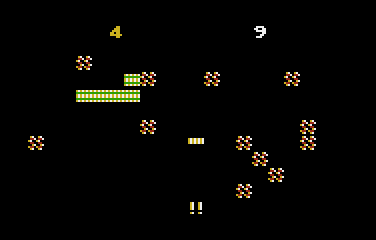Prototype using ANTIC 5

This way, it looked like the segments were moving faster than before.

The next issue to solve was the fact that there would be an obvious difference in the speed between 1 and 3 segments moving around. Instead of the FOR-NEXT loop with a variable upper limit, I used a counter that iterates over all 3 segments, and did nothing but a pause when it was time to manage an unexistant segment.

There is an array with the same max size of the caterpillar. This array works as a list of pointers to the screen memory of every segment of the caterpillar. There is another pointer that says which element has the head, and the body of the segments are following elements, up to the current lenght of the segment. This array works like a continuous ring. When the caterpillar advances one step in the screen, the current last element of the array is freed, but then it is used as the next position of the head. This structure is the backbone of the game, and it is the same that was used in Minipede game with only one segment all the time. In this game, if there are multiple segments in the screen, there are many heads in the array, and probably only one free cell available between them. This is important, because the segment that must be moved first is the one that it has that free element just before its head.

With that in mind, it was time to write the code for the splits. But how could I recognize which segment was hit by the gun? I had to add another buffer where I could register which segment is drawn in every screen position. This way, I only have to sequentially scan the pointers array starting from the corresponding head.

But not every hit becomes a split. There are two special cases: a hit in the head and a hit in the tail. Those hits just shrinks the segment in one piece. In the case of the head, it is also required to manage the direction of movement if it has just step down one row.

When the game was working OK, it was time to provide the bitmaps for the sprites. As two bytes were used for each game element, I needed 2x8=16 bytes. I kept the left half with the same ATASCII code as in Minipede because those bytes are hardcoded to check for colissions, so the right half was assigned to the next bytes in the table.

While I was testing for bugs, I found it could be easily defeated by clearing out the mushrooms of the playfield. I had to find a way to avoid that situation. The first one was to make harder to destroy the mushrooms, requiring at least two hits. The order I assigned the codes allowed me to add a second shape for the mushrooms, a semidestroyed one. Nice! But I also add a simple row validation, in order that if it gets empty, a new mushroom randomly appear in it. Besides that, mushroom appear when you hit a segment, when a caterpillar steps down one row and for every segment that goes away from the playfield.

This game was an interesting experiment for low resolution games in ANTIC mode 5. I'll think what other games could be implemented in this colorful graphics mode.

Get the DECIPEDE.ATR file and set it as drive 1 in a real Atari or emulator. Turn on the computer and the game should start after loading. A joystick in port 1 is required.

## The code

The abbreviated BASIC code is the following: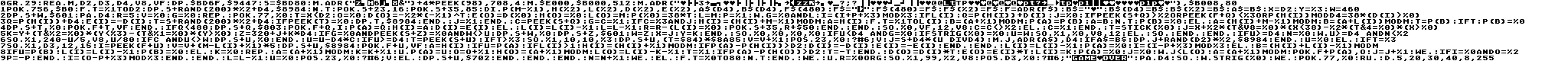The full and expanded BASIC listing is:

 ```graphics 29 ``` Initialize the screen in graphics mode 13+16. This is a ANTIC 5 screen without a text box. It has a resolution of 40x12 characters and allows 4 colors and a background. ```read m,d2,d3,d4,v8,vf data 5,20,30,40,8,255 ``` Assigns constant values. These are required to reduce the number of bytes in tokenized lines, because decimal numbers use 7 bytes and variables only 1 byte. Numbers in DATA use only the charactes in it. In the abbreviated version, the DATA line is at the end of the program. M is the max length of a caterpillar. ```dpoke \$BD6F,\$9447 ``` In a standard Atari running TurboBASIC XL, all the memory locations for graphics modes are fixed, so this DPOKE with constant values replaces the following expression: dpoke dpeek(560)+%3,((dpeek(88)+20) mod 256)*256+(7+\$40) This changes the first line from ANTIC mode 5 to mode 7 (text line like in graphics mode 2) and, at the same time, moves the screen data display pointer 20 bytes ahead, as mode 7 has requires only 20 bytes instead of 40. This line will be used for the score and the number of quits of the caterpillars. ```s=\$BD80 ``` This is the constant value of screen data pointer for graphics mode 13+16 (incluing those 20 bytes that won't be displayed). It's the same as s=dpeek(88) ```move adr("{data}")+4*peek(98),708,4 ``` Sets up the screen colors used for sprites and numbers: yellow, white, green and red. Background remains black. There are 8 bytes of data, 4 for PAL and 4 for NTSC, as both palettes are different, and one of the sets is chosen at runtime using the XL/XE's PALNTS register at memory address 98. ```move \$E000,\$B000,512 move adr("{data}"),\$B008,80 poke 756,\$B0 ``` Set up of the modified charset. It copies from the ROM the half of the charset where the digits and upper case letters are, and then replaces 10 chars, 8 bytes each. The 5 elements of the game are: player, bullet, caterpillar, full mushroom and half mushroom. The first 40 bytes are for the left half of the element and the remaining 40 bytes for the right half in the same order. ```for t=%1 to d2 dpoke s+rand(200)*%2+d4,\$8984 next t ``` Bestrews some mushrooms ramdomly over the playfield. The playfield is only 20x11 (skipping the first line and grouping bytes in pairs), and the bottom line will never have a mushroom. Only 20x10=200 places are candidates. ```poke s+23,16 poke s+35,85 ``` Displays the initial counters in the first line, each with a different color. ```dim p(m-%1),h(%2),l(%2),d(%2),e(%2),a\$(d4),b\$(d4),f\$(480) ``` Declares all the arrays and strings: P(): Pointers to each caterpillar piece on the screen. H(): Pointer to the pointer for the head of each segment. L(): Length of each segment. D(): Moving direction of each segment. E(): Temporary moving direction of each segment. A\$,B\$: Buffers to check if a playfield line is empty. F\$: Buffer to register which segment is in every corresponding screen location. ```f\$="{byte}" f\$(480)=f\$ f\$(%2)=f\$ f=adr(f\$) ``` Initializes the pieces' segment number buffer. ```b\$="{byte}" b\$(d4)=b\$ b\$(%2)=b\$ a\$=b\$ ``` Initializes both buffers to check for empty lines. One of them will receive a line every time a mushroom is removed. ```x=d2 y=%3 w=460 dpoke s+w,\$601 ``` Initial position on screen of the player. w=x+y*40+320, because the player can only move through the bottom 4 lines of the playfield. ```pause d4 ``` Adds a small pause before the start of the game. ```r=5 ``` Initial number of caterpillars that could go away. ```v=%0 ``` Initial score. ```g=%0 ``` Flag that indicates that the player was hit by the caterpillar. ```repeat ``` Main loop of the game: ``` poke 77,%0 ``` Resets ATRACT mode. ``` t=x ``` Initializes the first caterpillar. Depending of the current horizontal position of the player, the caterpillar will start the extreme top at the other side. Only the position of the head is initialized and the moving direction of it. Actually, both possible positions are in the first line of the screen data but never displayed over the score line, because, at the first move, the caterpillar will step down to the first displayed line of the playfield. ``` l=m ``` Initializes the total length of the caterpillars. ``` p=%1 ``` This flag says in which order must be moved the segments when there are more than one. It's tricky, because after the first split there are two caterpillars and the order remains the same as when there was only one. But the second split could be over any of the two segments. The sequence order must be reversed if the second split is over the original segment, because of the internal use of the screen pointers array. ``` while g=%0 and l ``` Round loop: ``` i=(i+p+%3) mod %3 ``` Increments the segment iterator. The order is 0, 1, 2, 0, 1, 2... ``` if l(i) ``` Check if the segment exists. Only segments with a length greater than zero has pieces on screen. ``` q=p(h(i))+d(i) ``` Finds where in screen is the next step of the segment. First, try the next horizontal position of the head based in the current movement direction. ``` j=%0 ``` Flag to check if the next step is in the same row. ``` if peek(s+q)>%2 or peek(f+q)<%3 or p(h(i)) mod d4=38*(d(i)>%0) ``` If next step is a mushroom or there is another caterpillar or it already is at the border: ``` q=p(h(i))+d4 ``` Sets next step as the same position at one row bellow. ``` e(i)=-d(i) ``` Temporary sets the moving direction to the other side. ``` t=s+rand(200)*%2+d4 if peek(t)=%0 dpoke t,\$8984 endif ``` Places a new mushroom in the playfield (if the randomly selected location is clear) every time the caterpillar moves down. ``` j=%1 ``` Sets the next row flag. ``` endif ``` ``` c=peek(s+q) ``` Retrieves the current element at the next head position. ``` g=c=%1 ``` Sets a flag if the player is at that position. If so, the caterpillar ate the player. ``` if c=%3 and j ``` If there is another caterpillar in the next row: ``` h(i)=(h(i)+m-%1) mod m a=h(i) for t=%1 to l(i) b=(a+%1) mod m p(a)=p(b) a=b next t p(b)=%0 ``` Current caterpillar becames stuck. All the pointers must be moved one place in the pointers array. The head now points to a new position in the array and the tail becames empty. ``` else ``` ``` a=(h(i)+m-%1) mod m ``` New index in the screen position's array for the head. It'll be one less tha the previous, and wraps to the end of the array if it is already at the first one. ``` b=(a+l(i)) mod m ``` Current index of the tail for it's screen position. ``` t=p(b) if t p(b)=%0 dpoke s+t,%0 poke f+t,vf endif ``` Remove the tail segment from screen, if it was already put. Then cleans the register in the array and the segments flags. ``` if q<480 dpoke s+q,\$803 poke f+q,i p(a)=q h(i)=a d(i)=e(i) ``` It adds the new head segment at the new position of the screen and records that position in the array. If the caterpillar has the full length, it'll be the same index as the released by the tail. This only happens if the new position is inside the playfield... ``` else l(i)=l(i)-%1 l=l-%1 r=r-(l(i)=%0) poke s+35,r+\$50 endif ``` If the new head position is out if the playfield, the length of the caterpillar is reduced by one, the head remains in the same place and decreases the number of lost rounds if it was the last piece of the caterpillar. ``` endif ``` ``` sound %0,vf,12,v8 ``` Starts the stepping sound in channel 0. ``` c=c<>%2 ``` Sets a flag for the bullet routine that indicates if the caterpillar hit the bullet in it's last movement. ``` else c=%1 endif ``` ``` t=stick(%0) j=x+%2*(t&v8=%0)*(x<38)-%2*(t&4=%0)*(x>%0) k=y+(t&%2=%0)*(y<%3)-(t&%1=%0)*(y>%0) z=320+j+k*d4 ``` Calculates the new location of the player based in its current location and the joystick position. ``` if g=%0 and peek(s+z)=%0 and w<>u dpoke s+w,%0 dpoke s+z,\$601 w=z x=j y=k endif ``` If the new location on screen is empty, this moves the player and sets its new location as the current one. ``` sound %0,%0,%0,%0 ``` Shuts up the stepping sound in channel 0. ``` if u ``` Checks if the current location of the bullet is out of the screen, enabling the player to fire. ``` if strig(%0)=%0 u=w sound %1,%0,v8,12 ``` If the fire button is pressed, sets the current location of the bullet at the player and starts a shot sound. ``` else sound endif endif ``` Shuts up the sound if the bullet is not on screen. ``` if u>=d4 ``` If there is a bullet in the playfield: ``` n=%0 ``` The bullet will step up two times on each game cycle. This initializes a counter. ``` while u>=d4 and n<%2 ``` If the bullet is still located in the playfield and this is one of the two times: ``` sound %1,240-u/5,v8,u/80 ``` Changes the shot sound based on the bullet's screen position. ``` if c and u<>w dpoke s+u,%0 endif ``` Remove the bullet form its current location. ``` u=u-d4*c ``` Sets the current location of the bullet one position up, unless it already was hit by the caterpilar's head in the last movement. ``` if u>=d4 t=peek(s+u) ``` If the new location of the bullet is still inside the playfield, chech first if there is another thing there. ``` if t>%3 ``` If there is a mushroom: ``` sound %1,10,10,%3 ``` Turns the shot sound by a hit into a mushroom sound. ``` dpoke s+u,(t=\$84)*\$8A85 ``` Turns a complete mushroom into a half mushroom, or remove a half mushroom. ``` v=v+%1 ``` Increases the score. ``` position 23,%0 ? #6;v ``` Prints the new score. ``` j=s+d4*(u div d4) move j,adr(a\$),d4 ``` Loads the screen line where the bullet hit the mushroom. ``` if a\$=b\$ dpoke j+rand(d2)*%2,\$8984 endif ``` Puts another mushroom if the line is empty. ``` u=%0 ``` Disables the bullet. ``` else ``` ``` if t=%3 ``` If there is a piece of the caterpillar: ``` sound %1,d3,12,15 ``` Turns the current shot sound into a caterpillar hit sound. ``` i=peek(f+u) ``` Gets the internal number of the caterpillar. ``` v=v+(m-l(i)+%1)*5 ``` Increases the score based on the current length of the caterpillar. ``` dpoke s+u,\$8984 ``` Replaces the segment by a mushroom in the playfield. ``` poke f+u,vf ``` Discards that position as a caterpillar in the buffer. ``` a=h(i) if u=p(a) ``` If the hit was in the head: ``` if l(i)>1 ``` If the segment has more than one piece: ``` h(i)=(h(i)+%1) mod m ``` Assign the next piece as the new head. ``` if p(a)-p(h(i))>d2 d(i)=-d(i) e(i)=-e(i) endif ``` If the old and the new head are in different playfield lines, change the movement direction to the opposite side. ``` endif ``` ``` l(i)=l(i)-%1 ``` Reduce the length of the segment by one. ``` p(a)=%0 ``` Clean the pointer to the old head. ``` i=(i-p+%3) mod %3 ``` Sets the segment iterator index as the previous segment in order to start with the new segment ni the next iteration. ``` else ``` ``` b=(h(i)+l(i)-%1) mod m ``` Gets the screen position on screen of the tail for the segment that was hit. ``` if u=p(b) l(i)=l(i)-%1 p(b)=%0 ``` If the hit was on the tail, just shrink the length by 1 and clean the pointer. ``` else ``` The hit was somewhere in the middle of a segment. ``` k=%0 repeat a=(a+%1) mod m k=k+%1 until p(a)=u ``` Finds which piece of the segment was hit, starting from the piece after the head. ``` o=o+%1 ``` Increments the number of active segments. ``` h(o)=(a+%1) mod m ``` Assigns the first piece of the new segmenta as another head. ``` l(o)=l(i)-k-%1 ``` Assigns the length of the new segment. ``` t=%1 if p(a)-p(h(o))>d2 t=-t endif ``` If the new segment's head is in a different row than the original's, flag to change the direction. ``` d(o)=d(i)*t e(o)=e(i)*t ``` Sets the direction for the new segment. ``` l(i)=k ``` Sets the new length of the original segment. ``` p(a)=%0 ``` Cleans the pointer where the hit splitted the segment. ``` j=%0 while j